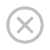# NumPy 数组连接

## 连接 NumPy 数组

### 实例

```import numpy as np

arr1 = np.array([1, 2, 3])

arr2 = np.array([4, 5, 6])

arr = np.concatenate((arr1, arr2))

print(arr)
```

### 实例

```import numpy as np

arr1 = np.array([[1, 2], [3, 4]])

arr2 = np.array([[5, 6], [7, 8]])

arr = np.concatenate((arr1, arr2), axis=1)

print(arr)
```

## 使用堆栈函数连接数组

### 实例

```import numpy as np

arr1 = np.array([1, 2, 3])

arr2 = np.array([4, 5, 6])

arr = np.stack((arr1, arr2), axis=1)

print(arr)
```

## 沿行堆叠

NumPy 提供了一个辅助函数：hstack() 沿行堆叠。

### 实例

```import numpy as np

arr1 = np.array([1, 2, 3])

arr2 = np.array([4, 5, 6])

arr = np.hstack((arr1, arr2))

print(arr)
```

## 沿列堆叠

NumPy 提供了一个辅助函数：vstack() 沿列堆叠。

### 实例

```import numpy as np

arr1 = np.array([1, 2, 3])

arr2 = np.array([4, 5, 6])

arr = np.vstack((arr1, arr2))

print(arr)
```

## 沿高度堆叠（深度）

NumPy 提供了一个辅助函数：dstack() 沿高度堆叠，该高度与深度相同。

### 实例

```import numpy as np

arr1 = np.array([1, 2, 3])

arr2 = np.array([4, 5, 6])

arr = np.dstack((arr1, arr2))

print(arr)
```